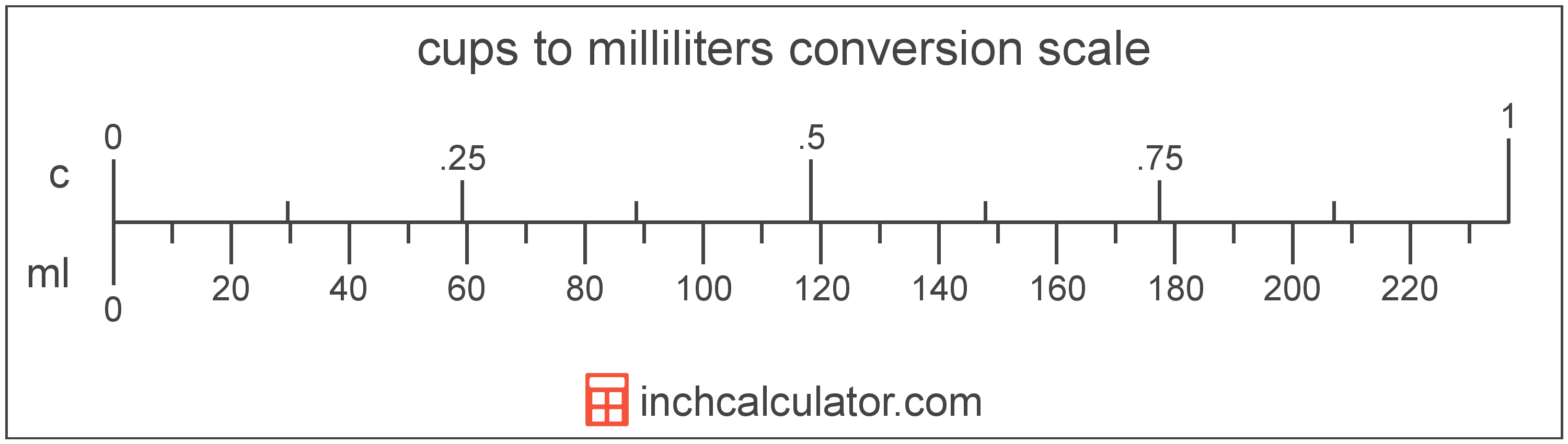# Milliliters to Cups Converter

Enter the volume in milliliters below to get the value converted to cups.

Results in Cups:1 mL = 0.004227 c
1 mL < 1/16 c

Do you want to convert cups to milliliters?

## How to Convert Milliliters to Cups

To convert a measurement in milliliters to a measurement in cups, multiply the volume by the following conversion ratio: 0.004227 cups/milliliter.

Since one milliliter is equal to 0.004227 cups, you can use this simple formula to convert:

cups = milliliters × 0.004227

The volume in cups is equal to the volume in milliliters multiplied by 0.004227.

For example, here's how to convert 500 milliliters to cups using the formula above.
cups = (500 mL × 0.004227) = 2.113376 c## What Is a Milliliter?

The milliliter is a unit of volume equal to 1 cubic centimeter, 1/1,000 of a liter, or about 0.061 cubic inches.

The milliliter is an SI unit of volume in the metric system. In the metric system, "milli" is the prefix for thousandths, or 10-3. A milliliter is sometimes also referred to as a millilitre. Milliliters can be abbreviated as mL, and are also sometimes abbreviated as ml or mℓ. For example, 1 milliliter can be written as 1 mL, 1 ml, or 1 mℓ.

Since one milliliter is equivalent to one cubic centimeter, milliliters are sometimes expressed using the abbreviation for a cubic centimeter (cc) for things such as medical dosages or engine displacements.

## What Is a Cup?

The cup is a unit of volume equal to 16 tablespoons or 8 fluid ounces. The cup should not be confused with the metric cup or the teacup, which are different units of volume.

One cup is equal to 236.588 milliliters, but in nutrition labeling, one cup is defined as 240 milliliters. To further confuse things, a metric cup is equal to 250 mL, while in Japan, a cup is equivalent to only 200 mL.

The cup is a US customary unit of volume. Cups can be abbreviated as c, and are also sometimes abbreviated as C. For example, 1 cup can be written as 1 c or 1 C.

## Milliliter to Cup Conversion Table

Table showing various milliliter measurements converted to cups.
Milliliters Cups
1 mL 0.004227 c
2 mL 0.008454 c
3 mL 0.01268 c
4 mL 0.016907 c
5 mL 0.021134 c
6 mL 0.025361 c
7 mL 0.029587 c
8 mL 0.033814 c
9 mL 0.038041 c
10 mL 0.042268 c
20 mL 0.084535 c
30 mL 0.126803 c
40 mL 0.16907 c
50 mL 0.211338 c
60 mL 0.253605 c
70 mL 0.295873 c
80 mL 0.33814 c
90 mL 0.380408 c
100 mL 0.422675 c
200 mL 0.845351 c
300 mL 1.268 c
400 mL 1.6907 c
500 mL 2.1134 c
600 mL 2.5361 c
700 mL 2.9587 c
800 mL 3.3814 c
900 mL 3.8041 c
1,000 mL 4.2268 c

## References

1. National Institute of Standards and Technology, Specifications, Tolerances, and Other Technical Requirements for Weighing and Measuring Devices, Handbook 44 - 2019 Edition, https://nvlpubs.nist.gov/nistpubs/hb/2019/NIST.HB.44-2019.pdf
2. U.S. Food & Drug Administration, Guidance for Industry: Guidelines for Determining Metric Equivalents of Household Measures, https://www.fda.gov/regulatory-information/search-fda-guidance-documents/guidance-industry-guidelines-determining-metric-equivalents-household-measures